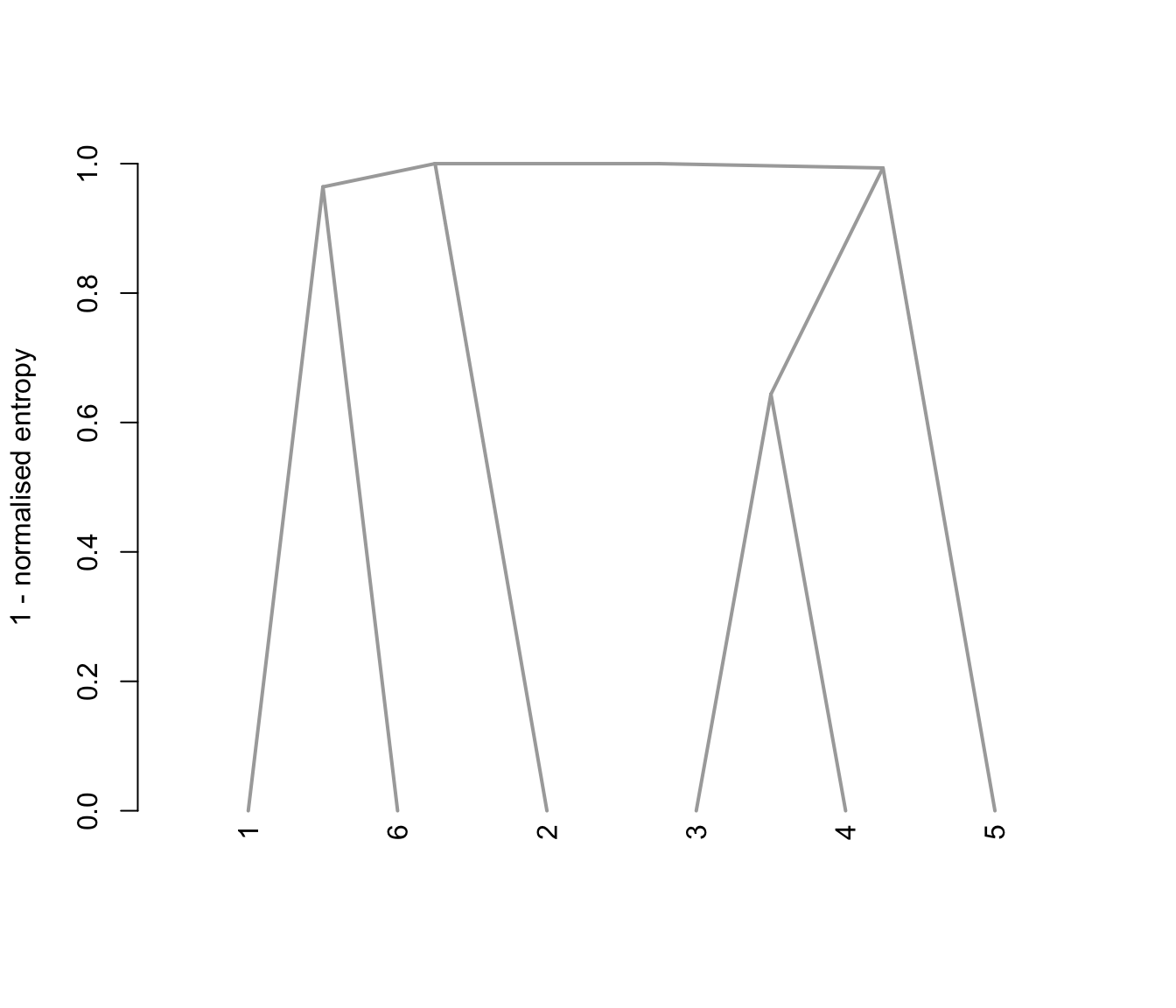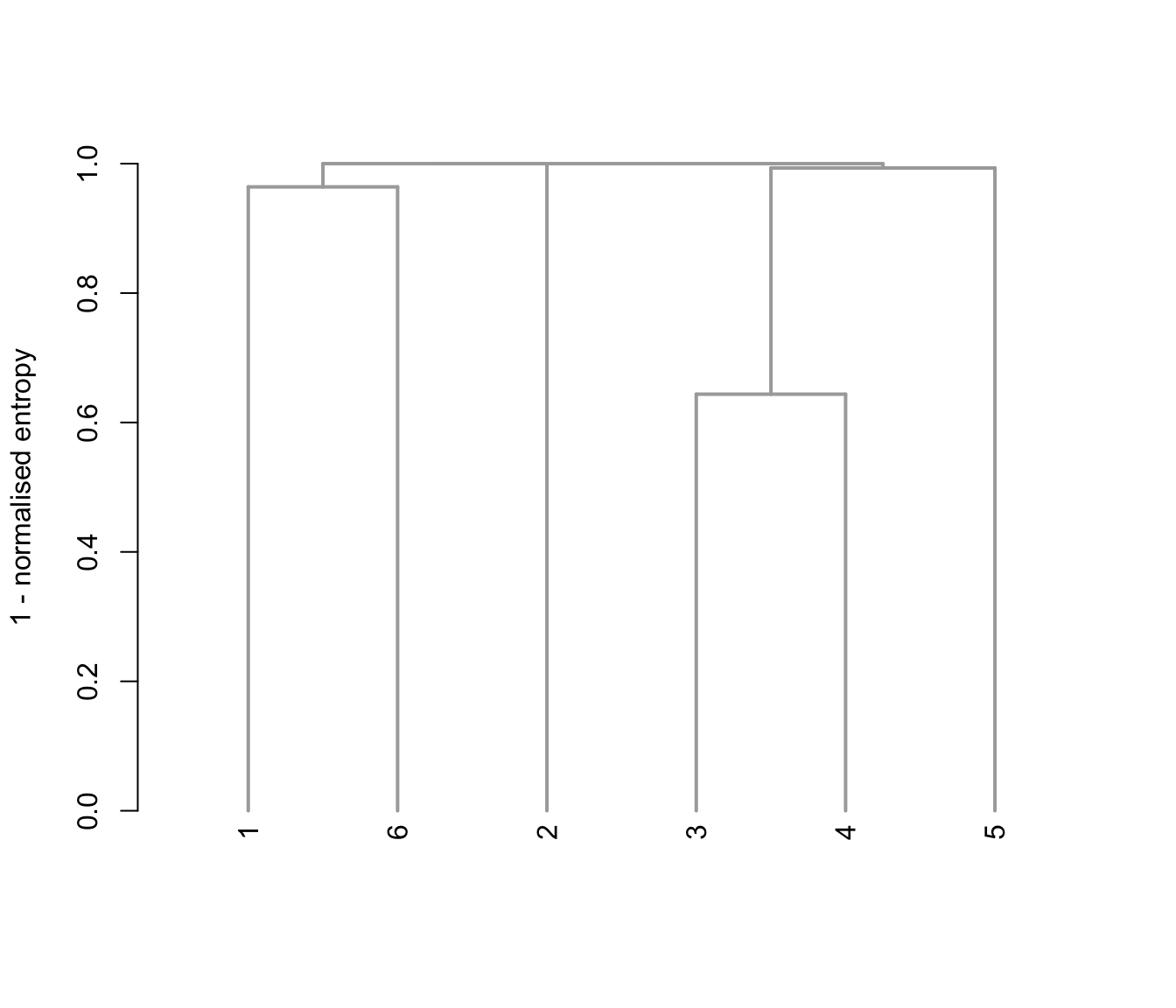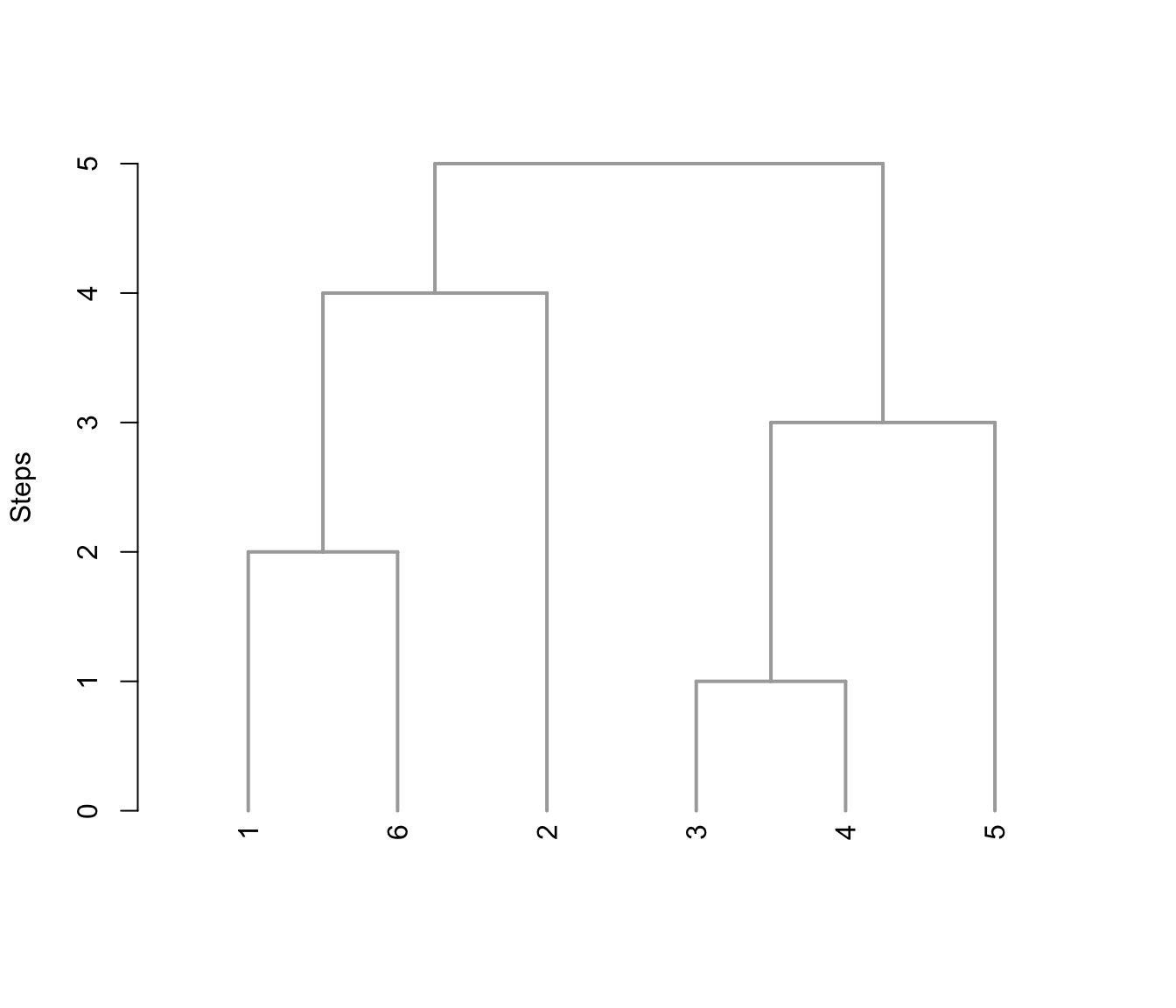The method implemented in clustCombi can be used for combining Gaussian mixture components for clustering. This provides a hierarchical structure which can be graphically represented as a tree.

combiTree(object, type = c("triangle", "rectangle"),
yaxis = c("entropy", "step"),
edgePar = list(col = "darkgray", lwd = 2),
...)

Arguments

object

An object of class 'clustCombi' resulting from a call to clustCombi.

type

A string specifying the dendrogram's type. Possible values are "triangle" (default), and "rectangle".

yaxis

A string specifying the quantity used to draw the vertical axis. Possible values are "entropy" (default), and "step".

edgePar

A list of plotting parameters. See dendrogram.

...

Further arguments passed to or from other methods.

Value

The function always draw a tree and invisibly returns an object of class 'dendrogram' for fine tuning.

L. Scrucca

Examples

# \donttest{
data(Baudry_etal_2010_JCGS_examples)
output <- clustCombi(data = ex4.1)
combiTree(output)combiTree(output, type = "rectangle")combiTree(output, yaxis = "step")combiTree(output, type = "rectangle", yaxis = "step")# }